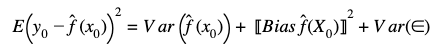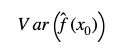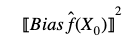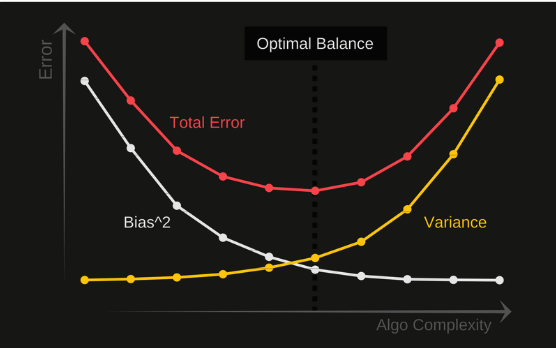The interesting property of a machine learning model is its capacity to predict or categorize new unseen data (data that was not used in training the model). For this reason the important measure is the MSE error with test data, which is denominated as test MSE. The goal is to choose a model where the test MSE is the lowest across other models.

The bias variance tradeoff  is generated when at some point if we increase the bias of the model by creating additional features, the variance of the model increases too (overfitting), and on the other hand if the model is too simple (has very few parameters),  it will have high bias and low variance (under fitting).

It is necessary to find the right balance between bias and variance without overfitting and under fitting the data. The prediction error in a Supervised machine learning algorithm can be divided into three different parts:

• Bias Error
• Variance Error
• Irreducible Error

First we will write the equation which breaks these three factors:Variance ErrorThe first term on the right hand side is the variance of the estimation across many testing sets. This measures the average model deviation among different testing data. In particular, a model with high variance is suggestive that it is overfit to the training data. In this scenario, the model is capturing the noise of the training dataset but it is poor for new data.

Bias ErrorThe squared bias characterizes the difference between the averages of the estimate and the true values. A model that has high bias, would not capture the underlying behavior of the true functional form well. One example of this case is when a linear regression is used to fit a model with a non-linearity relationship between their features.

Irreducible error

Var(∈)

This term represents the minimum lower bound for the test MSE. This error cannot be reduced regardless what algorithm is used. This error reflects the influence that unknown variables have between the interaction of the features and the target variable.

Bias and variance moves in different direction and the relative rate of change between these two factors determines whether the expected test MSE increases or decreases.

In the plot below we show the trade-off between bias and variance. At first time, as flexibility increase the bias tend to drop quickly, faster than the increase in variance generating a reduction on the test MSE of the model (Total Error). However, as flexibility increases further, there is less reduction in bias and the variance rapidly increase, causing model overfitting.

The goal of any supervised machine learning algorithm is to achieve low bias and low variance, and all models need a well balance between these factors. For this reason is necessary to understand both factors to reach the optimal point that consist in the lower bias-variance.